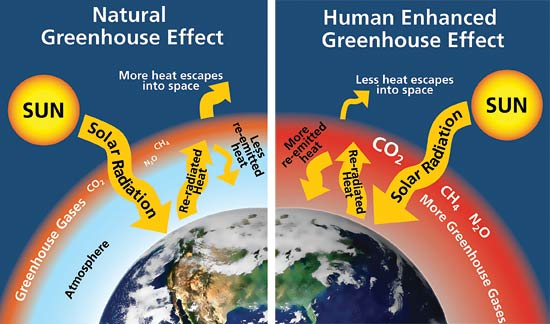Climate Change Assignment
starstarstarstarstarstarstarstarstarstar
2 (1 rating)
by Eric Cale
| 13 Questions
Note from the author:
This assignment pulls data (CO2 levels, sea levels, temperature, etc) from climate.nasa.gov and asks students to analyze the graphs to see what kind of evidence we have for climate change. It also asks students to summarize what they understand about climate change based on this data. It is aligned to Indiana Science Standard 8.ESS.1.
The following graph shows the average "Temperature Anomaly" (temperature difference) from 1880-Present. This graph was taken from climate.nasa.gov.1
2
The average global temperature has been on a rising trend since the 1960s.
True
False
2
2
In the last 100 years, about how much has the average global temperature changed? (To the nearest tenth)
3
2
What do you suppose might be the cause of this change in the average global temperature?
Below is a diagram that describes how the greenhouse effect works. Use this to answer the next two questions.4
2
What do greenhouse gases do?
Block the Sun's radiation from entering Earth's atmosphere, lowering Earth's temperature.
Help to trap the Sun's radiation into the Earth's atmosphere, raising Earth's temperature.
Block the Sun's radiation from entering Earth's atmosphere, raising Earth's temperature.
Help to trap the Sun's radiation into the Earth's atmosphere, lowering Earth's temperature.
5
6
Explain what happens when there is a lot of extra greenhouse gases in our atmosphere. Explain what happens when there's not enough greenhouse gases in our atmosphere. Based on those answers, are greenhouse gases inherently good or bad? Explain.
Carbon dioxide (formula shown below) is a greenhouse gas. The graphs below shows how many molecules of carbon dioxide have been in a million molecules of our atmosphere over a number of years (taken from climate.nasa.gov). Use these graphs to answer the next two questions.6
2
How much more carbon dioxide was in our atmosphere in 2017 than in 2006 (answer in ppm)?
7
4
What was the highest historical carbon dioxide level (2nd graph)? What is our carbon dioxide level now (1st graph)? Why is this information useful to us?
The graphs below show the amount of ice in the Arctic Region (by area) and in the Anarctic Region (by mass) over a number of years. Use these graphs to answer the next two questions.8
2
Which best describes the amount of ice in the Arctice and Antarctic regions of Earth?
It is increasing.
It is remaining the same.
It is decreasing.
9
2
What do you suppose is causing this general trend in the amount of ice on Earth's poles?
The graph below shows the average sea level change for the last 24 years, measured in millimeters. Use this graph to answer the next two questions.10
2
About how much as the Earth's average sea level changed in the since 1993 (in mm)? Has it increased or decreased?
11
2
What do you suppose is causing this change in the Earth's average sea level?
12
2
Do some research. What does "global warming" refer to? What does "climate change" refer to?
13
5
Write 4-6 sentences that summarizes all of the information in this assigment (global temperatures, amount of ice, sea level, greenhouse gases, amount of carbon dioxide, global warming, and climate change). Be very descriptive.